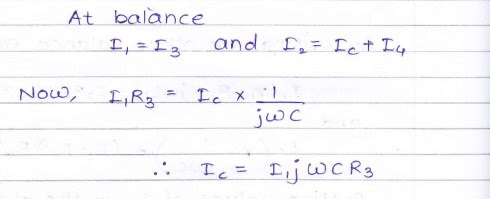Anderson's Bridge is the modification of Maxwell's inductance-capacitance bridge. In Anderson's bridge, a standard capacitor is used for the measurement of self-inductance. The main advantage of this method is that it can be used for the wide range of self-inductance measurement.

The following figure shows Anderson's bridge for the balance conditions.Let,

• L1 = Self-inductance to be measured,

• R1 = resistance of self-inductor,

• r1 = resistance connected in series with self-inductor,

• r, R2, R3, Ra = known non-inductive resistances,

• C = fixed standard capacitor.

At balance,1)      In Anderson's bridge, it is very easy to obtain the balance point as compared to Maxwell’s bridge.

2)      In this bridge, a fixed standard capacitor is used therefore there is no need of costly variable capacitor.

3)      This method is very accurate for measurement of capacitance in terms of inductance.

1) It is more complex as compared with Maxwell’s inductance bridge. It has more parts and hence complex in setting up and manipulate. The balance equations of Anderson's bridge are quite complex and much more tedious.

2) An additional junction point increases the difficulty of shielding the bridge.

You may also like:

1.2.